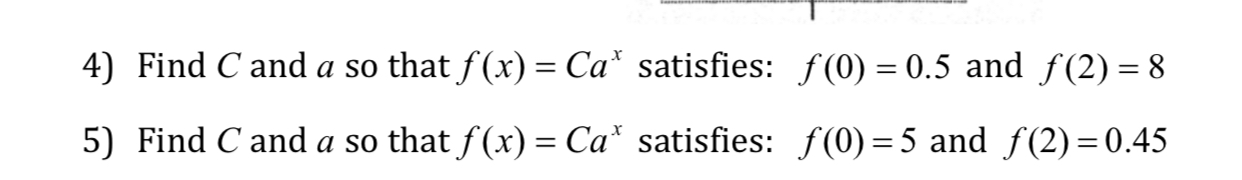# 4) Find C and a so thatf(x Ca satisfies: f(0) 0.5 and f(2)-8 5) Find Canda so that f(x)-Cax satisfies: f(0) = 5 and f(2)=0.45

Questionhelp_outlineImage Transcriptionclose4) Find C and a so thatf(x Ca satisfies: f(0) 0.5 and f(2)-8 5) Find Canda so that f(x)-Cax satisfies: f(0) = 5 and f(2)=0.45 fullscreen

### Want to see this answer and more?

Experts are waiting 24/7 to provide step-by-step solutions in as fast as 30 minutes!*